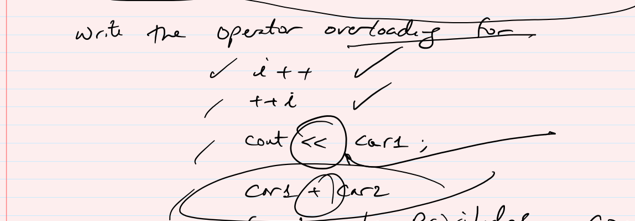# Question & Answer: (C++) I feel confused about the operator overloading. Could you write an easy and simple program to explain it? Thanks…..

(C++) I feel confused about the operator overloading. Could you write an easy and simple program to explain it? ThanksDon't use plagiarized sources. Get Your Custom Essay on
Question & Answer: (C++) I feel confused about the operator overloading. Could you write an easy and simple program to explain it? Thanks…..
GET AN ESSAY WRITTEN FOR YOU FROM AS LOW AS \$13/PAGE

In C++ the overloading applies to operators also. That means operators can be extended not only with built-in functions but also in classes too. We can provide operator to a class by overloading the built-in operator.Here I’m writing c++ program that overloads the pre-increment and post-increment operators.In case of pre-increment operator value first attribute is incremented and the reference to result is returned whereas in the post-increment operator,first a local copy is saved, and the attribute is incremented and finally reference to the local copy is returned.

#include <iostream>
using namespace std;

class Integer {
private:
int value;
public:
Integer(int v) : value(v) { }
Integer operator++();
Integer operator++(int);
int getValue() {
return value;
}
};

Integer Integer::operator++() //pre-increment
{
value++;
return *this;
}

Integer Integer::operator++(int) // Post-increment
{
const Integer old(*this);
++(*this);
return old;
}

int main()
{
Integer i(10);

cout << “Post Increment Operator” << endl;
cout << “Integer++ : ” << (i++).getValue() << endl;
cout << “Pre Increment Operator” << endl;
cout << “++Integer : ” << (++i).getValue() << endl;
}
\$ a.out
Post Increment Operator
Integer++ : 10
Pre Increment Operator
Integer++ : 12